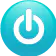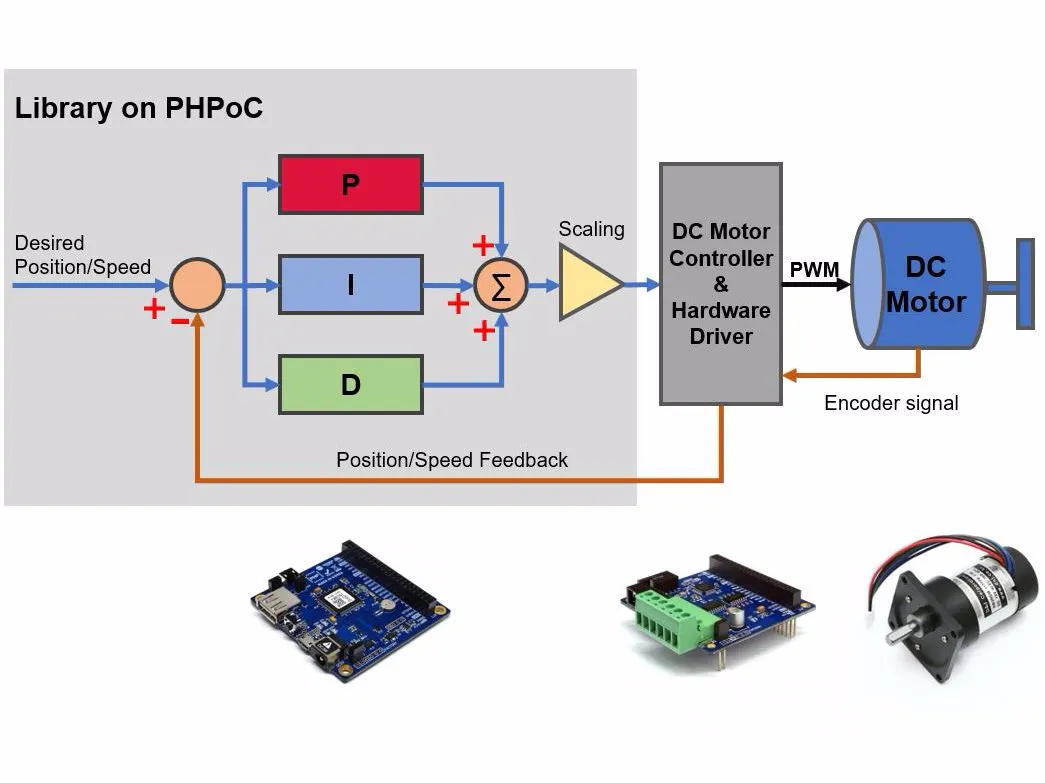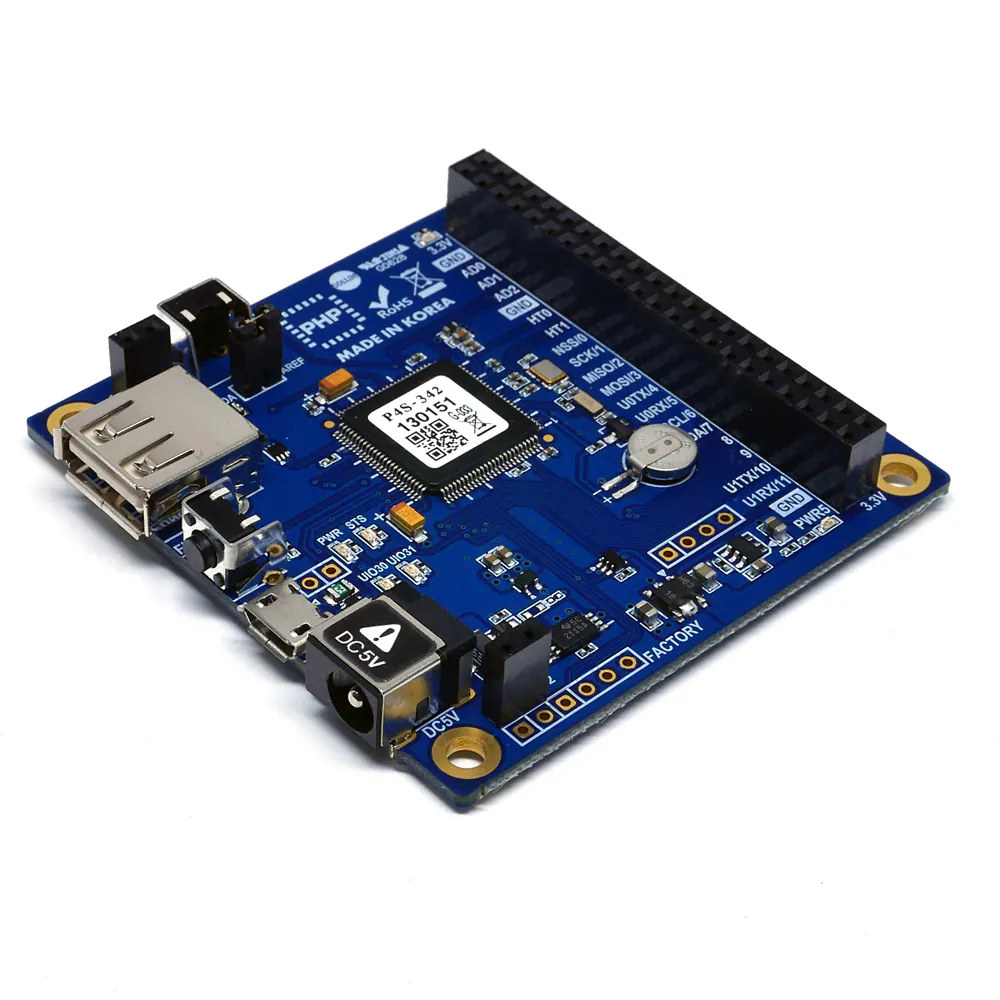# PID Controller, Auto-tuning Library And Example For DC Motor

This provides libraries and examples code of controlling position and speed of DC motor using PID controller and auto-tuning.

BeginnerFull instructions provided10,957## Things used in this project

### Hardware componentsPHPoC Blue You can use PHPoC Black instead
×1PHPoC DC Motor Controller (S-type or T-type)
×1
 DC Motor with Encoder
×1

## Code

PHP
Examples of speed control
```<?php
include_once "/lib/sd_340.php";
include_once "/lib/sd_spc.php";
include_once "vc_pid.php";

function dc_get_speed(\$sid, \$dc_id)
{
\$enc_period = (int)spc_request_dev(\$sid, "dc\$dc_id enc get period");

if(!\$enc_period)
\$speed = 1;
else
\$speed = 1000000 / \$enc_period;

return \$speed;
}

\$sid = 14;
\$dc_id = 1;
\$pwm_period = 10000; //10000 us

st_free_setup(0, "us");

spc_reset();
spc_sync_baud(460800);
spc_request_dev(\$sid, "dc\$dc_id pwm set period \$pwm_period");
spc_request_dev(\$sid, "dc\$dc_id enc set psr 64");
spc_request_dev(\$sid, "dc\$dc_id pwm set decay slow");
spc_request_dev(\$sid, "dc\$dc_id lpf set freq 5000");

/*-----------PID parameters-----------*/
\$pid = array(0, 0, 0, 0, 0, 0, 0, 0, 0, 0, 0, 0);

//\$pid[INDEX_KP_GAIN]	= 0; // manually set by user or automatically set by auto-tuning function
//\$pid[INDEX_KI_GAIN]	= 0; // manually set by user or automatically set by auto-tuning function
//\$pid[INDEX_KD_GAIN]	= 0; // manually set by user or automatically set by auto-tuning function
\$pid[INDEX_INT_TERM]	= 0; // initial value
\$pid[INDEX_PRE_ERROR]	= 0; // initial value
\$pid[INDEX_INT_MAX]  	= 0; // disble limit
\$pid[INDEX_INT_MIN]	    = 0; // disble limit
\$pid[INDEX_PRE_TIME]    = st_free_get_count(0); //current time
\$pid[INDEX_SAMPLE_TIME]	= 10000; // in micro-second
\$pid[INDEX_PID_TYPE]	= PID_TYPE_SPEED; // speed control
\$pid[INDEX_PID_SCALE]	= 8; // depending on motor, should test manually to have the best value
\$pid[INDEX_PWM_PERIOD]	= \$pwm_period;

\$setpoint = 3000; //desired speed

/*----------- PID auto-tuning-------------- */
dc_pid_tune_start();
while(!dc_pid_tune_loop(\$sid, \$dc_id, \$pid))
usleep(10000);

/*----------- loop -----------*/
\$i = 0;
while(\$i < 3000)
{
if(\$i == 0)
\$setpoint = 3000;
else if(\$i == 1000)
\$setpoint = 4000;
else if(\$i == 2000)
\$setpoint = 1000;

dc_pid_loop(\$sid, \$dc_id, \$setpoint, \$pid);

\$speed = dc_get_speed(\$sid, \$dc_id);
echo "\$speed\r\n";
\$i++;

usleep(1000);
}

spc_request_dev(\$sid, "dc\$dc_id pwm set width 0");

?>
```

PHP
Example of position control
```<?php
include_once "/lib/sd_340.php";
include_once "/lib/sd_spc.php";
include_once "vc_pid.php";

\$sid = 14;
\$dc_id = 1;
\$pwm_period = 10000; //10000 us

st_free_setup(0, "us");

spc_reset();
spc_sync_baud(460800);
spc_request_dev(\$sid, "dc\$dc_id pwm set period \$pwm_period");
spc_request_dev(\$sid, "dc\$dc_id enc set psr 64");
spc_request_dev(\$sid, "dc\$dc_id pwm set decay slow");
spc_request_dev(\$sid, "dc\$dc_id lpf set freq 5000");

/*----------- check enc pol-------------- */
\$pwm = 0; \$pos = 0;
while(!\$pos)
{
\$pwm += 10;
spc_request_dev(\$sid, "dc\$dc_id pwm set width \$pwm");
\$pos = (int)spc_request_dev(\$sid, "dc\$dc_id enc get pos");
usleep(1000);
}

if(\$pos < 0)
spc_request_dev(\$sid, "dc\$dc_id enc set pol -");

spc_request_dev(\$sid, "dc\$dc_id pwm set width 0");

/*-----------PID parameters-----------*/
\$pid = array(0, 0, 0, 0, 0, 0, 0, 0, 0, 0, 0, 0);

//\$pid[INDEX_KP_GAIN]	= 0; // manually set by user or automatically set by auto-tuning function
//\$pid[INDEX_KI_GAIN]	= 0; // manually set by user or automatically set by auto-tuning function
//\$pid[INDEX_KD_GAIN]	= 0; // manually set by user or automatically set by auto-tuning function
\$pid[INDEX_INT_TERM]	= 0; // initial value
\$pid[INDEX_PRE_ERROR]	= 0; // initial value
\$pid[INDEX_INT_MAX]  	= 6000; // recommended vaule <= 100% of pwm width for position control
\$pid[INDEX_INT_MIN]	    = -6000; // opposite of upper limit
\$pid[INDEX_PRE_TIME]    = st_free_get_count(0); //current time
\$pid[INDEX_SAMPLE_TIME]	= 10000; // in micro-second
\$pid[INDEX_PID_TYPE]	= PID_TYPE_POS; // position control
\$pid[INDEX_PID_SCALE]	= 16; // depending on motor, should test manually to have the best value
\$pid[INDEX_PWM_PERIOD]	= \$pwm_period;

\$setpoint = 2000; //desired position

/*----------- PID auto-tuning-------------- */
dc_pid_tune_start();
while(!dc_pid_tune_loop(\$sid, \$dc_id, \$pid))
usleep(10000);

spc_request_dev(\$sid, "dc\$dc_id enc set pos 0");

/*----------- loop -----------*/
\$i = 0;
while(\$i < 3000)
{
if(\$i == 0)
\$setpoint = 2000;
else if(\$i == 1000)
\$setpoint = 3000;
else if(\$i == 2000)
\$setpoint = 1000;

dc_pid_loop(\$sid, \$dc_id, \$setpoint, \$pid);

\$pos = (int)spc_request_dev(\$sid, "dc\$dc_id enc get pos");
echo "\$pos \$setpoint\r\n";
\$i++;

usleep(1000);
}

spc_request_dev(\$sid, "dc\$dc_id pwm set width 0");

?>
```

### vc_pid.php

PHP
PID library (including auto-tuning)
```<?php

define("INDEX_KP_GAIN",		0); // index of proportional gain in pid array
define("INDEX_KI_GAIN",		1); // index of integral gain in pid array
define("INDEX_KD_GAIN",		2); // index of differential gain in pid array
define("INDEX_INT_TERM",	3); // index of integral term in pid array
define("INDEX_PRE_ERROR",	4); // index of last error in pid array
define("INDEX_INT_MAX",	    5); // index of maximum integral in pid array
define("INDEX_INT_MIN",	    6); // index of minimum integral in pid array
define("INDEX_PRE_TIME",	7); // index of last calculated time in pid array
define("INDEX_SAMPLE_TIME",	8); // index of sampling time
define("INDEX_PID_TYPE", 	9); // index of pid type: speed: PID_TYPE_SPEED or position: PID_TYPE_POS
define("INDEX_PID_SCALE", 	10); // index of pid scale factor
define("INDEX_PWM_PERIOD", 	11); // index of PWM period

define("PID_TYPE_NONE",  0);
define("PID_TYPE_POS",   1);
define("PID_TYPE_SPEED", 2);

define("INT_OVERFLOW",	 0x7fffffffffffffff);
define("INT_UNDERFLOW",	 0x8000000000000000);

/* PID tuning state machine */
define("PID_TUNE_STATE_IDLE",   0);
define("PID_TUNE_STATE_START",  1);
define("PID_TUNE_STATE_WAIT",   2);
define("PID_TUNE_STATE_LOOP",   3);
define("PID_TUNE_STATE_END",    4);

\$pid_tune_state 		= PID_TUNE_STATE_START;

\$pid_tune_input 		= 0;
\$pid_tune_base_input	= 0;
\$pid_tune_step 			= 0;
\$pid_tune_setpoint 		= 0;
\$pid_tune_pre_feedback 	= 0;
\$pid_tune_cur_feedback 	= 0;

\$pid_tune_change_dir 	= 0; //0: initial, 1: go up, -1 go down
\$pid_tune_max_sum 		= 0.0;
\$pid_tune_min_sum 		= 0.0;
\$pid_tune_max_count 	= 0;
\$pid_tune_min_count 	= 0;
\$pid_tune_noiseband 	= 2;
\$pid_tune_start_time 	= 0;
\$pid_tune_end_time 		= 0;
\$pid_tune_wait_count 	= 0;

function dc_pid_compute(\$target, \$feedback, &\$dc_pid)
{
\$cur_time = st_free_get_count(0);
\$sample_time = (\$cur_time - \$dc_pid[INDEX_PRE_TIME]) / 1000000.0;

if(\$sample_time <=0)
return 0;

\$Kp = \$dc_pid[INDEX_KP_GAIN];
\$Ki = \$dc_pid[INDEX_KI_GAIN];
\$Kd = \$dc_pid[INDEX_KD_GAIN];
\$I_term = \$dc_pid[INDEX_INT_TERM];
\$pre_err = \$dc_pid[INDEX_PRE_ERROR];

\$cur_err = \$target - \$feedback;

/* proportional term computation */
\$P_term = \$Kp * \$cur_err;

/* integral term computation */
if((float)\$Ki == 0.0)
\$I_term = 0;
else
{
\$partition = \$Ki * \$cur_err * \$sample_time;

if(\$partition > 0)
\$partition  = ceil(\$partition);
else
\$partition  = floor(\$partition);

\$I_term += \$partition;

/*
// Check  integral overflow
if(\$I_term > 0 && \$partition < 0 && \$dc_pid[INDEX_INT_TERM] < 0)
\$I_term = INT_UNDERFLOW;

else if(\$I_term < 0 && \$partition > 0 && \$dc_pid[INDEX_INT_TERM] > 0)
\$I_term = INT_OVERFLOW;
*/

// Check integral bound
if(\$dc_pid[INDEX_INT_MAX] != 0 &&(\$I_term > \$dc_pid[INDEX_INT_MAX]))
\$I_term = \$dc_pid[INDEX_INT_MAX];

if(\$dc_pid[INDEX_INT_MIN] != 0 &&(\$I_term < \$dc_pid[INDEX_INT_MIN]))
\$I_term = \$dc_pid[INDEX_INT_MIN];
}

/* differential term computation */
\$D_term = \$Kd * (\$cur_err - \$pre_err) / \$sample_time;

\$output = round(\$P_term + \$I_term + \$D_term);

\$dc_pid[INDEX_INT_TERM] = \$I_term;
\$dc_pid[INDEX_PRE_ERROR] = \$cur_err;
\$dc_pid[INDEX_PRE_TIME] = \$cur_time;

return (int)\$output;
}

function dc_pid_loop(\$sid, \$dc_id, \$target, &\$pid)
{
\$cur_time = st_free_get_count(0);
\$sample_time = \$cur_time - \$pid[INDEX_PRE_TIME];

if(\$sample_time >= \$pid[INDEX_SAMPLE_TIME])
{
if(\$pid[INDEX_PID_TYPE] == PID_TYPE_POS)
{
\$cur_pos = (int)spc_request_dev(\$sid, "dc\$dc_id enc get pos");

\$pwm_width = (int)dc_pid_compute(\$target, \$cur_pos, \$pid) / \$pid[INDEX_PID_SCALE];

if(\$pwm_width >= 0)
\$dir = "+";
else
{
\$dir = "-";
\$pwm_width *= -1;
}

if(\$target == \$cur_pos)
{
\$pid[INDEX_INT_TERM] = 0;
\$pwm_width = 0;
}

spc_request_dev(\$sid, "dc\$dc_id pwm set dir \$dir");
spc_request_dev(\$sid, "dc\$dc_id pwm set width \$pwm_width");
}
else if(\$pid[INDEX_PID_TYPE] == PID_TYPE_SPEED)
{
\$enc_period = (int)spc_request_dev(\$sid, "dc\$dc_id enc get period");

if(!\$enc_period)
\$cur_speed = 1;
else
\$cur_speed = 1000000 / \$enc_period;

\$pwm_width = (int)dc_pid_compute(\$target, \$cur_speed, \$pid) / \$pid[INDEX_PID_SCALE];

if(\$target == \$cur_speed)
return;

if(\$pwm_width < 0)
\$pwm_width = 0;

spc_request_dev(\$sid, "dc\$dc_id pwm set width \$pwm_width");
}
}
}

function dc_pid_tune_start()
{
global \$pid_tune_state;
\$pid_tune_state = PID_TUNE_STATE_START;
}

function dc_pid_tune_loop(\$sid, \$dc_id, &\$pid)
{
global \$pid_tune_state;

global \$pid_tune_input;
global \$pid_tune_base_input;
global \$pid_tune_step;
global \$pid_tune_setpoint;
global \$pid_tune_pre_feedback;
global \$pid_tune_cur_feedback;

global \$pid_tune_change_dir;
global \$pid_tune_max_sum;
global \$pid_tune_min_sum;
global \$pid_tune_max_count;
global \$pid_tune_min_count;
global \$pid_tune_noiseband;
global \$pid_tune_start_time;
global \$pid_tune_end_time;

global \$pid_tune_wait_count;

switch(\$pid_tune_state)
{
case PID_TUNE_STATE_START:
//echo "PID: Tuning...\r\n";

\$pwm_period = \$pid[INDEX_PWM_PERIOD];

if(\$pid[INDEX_PID_TYPE] == PID_TYPE_POS)
{
\$pid_tune_base_input = 0;
\$pid_tune_step = \$pwm_period * 3 / 10; /* 30% */
}
else if(\$pid[INDEX_PID_TYPE] == PID_TYPE_SPEED)
{
\$pid_tune_base_input = \$pwm_period * 4 / 10; /* 40% */
\$pid_tune_step = \$pwm_period / 10; /* 10% */
}
else
return false;

\$pid_tune_input = \$pid_tune_base_input;

spc_request_dev(\$sid, "dc\$dc_id pwm set width \$pid_tune_input");

\$pid_tune_wait_count = 500000 / \$pid[INDEX_SAMPLE_TIME]; // 500000 us
\$pid_tune_state = PID_TUNE_STATE_WAIT;
return false;

case PID_TUNE_STATE_WAIT:
/* wait to let process settle to a steady state */
if(\$pid_tune_wait_count--)
return false;

if(\$pid[INDEX_PID_TYPE] == PID_TYPE_POS)
\$pid_tune_setpoint = (int)spc_request_dev(\$sid, "dc\$dc_id enc get pos");
else if(\$pid[INDEX_PID_TYPE] == PID_TYPE_SPEED)
{
\$enc_period = (int)spc_request_dev(\$sid, "dc\$dc_id enc get period");

if(!\$enc_period)
\$enc_pps = 1;
else
\$enc_pps = 1000000 / \$enc_period;

\$pid_tune_setpoint = \$enc_pps;
}
else
return false;

\$pid_tune_pre_feedback = \$pid_tune_setpoint;

\$pid_tune_change_dir = 0;
\$pid_tune_max_sum = 0.0;
\$pid_tune_min_sum = 0.0;
\$pid_tune_max_count = 0;
\$pid_tune_min_count = 0;
\$pid_tune_noiseband = 4;
\$pid_tune_start_time = 0;
\$pid_tune_end_time = 0;

\$pid_tune_input = \$pid_tune_base_input + \$pid_tune_step;

\$pid_tune_state = PID_TUNE_STATE_LOOP;
return false;

case PID_TUNE_STATE_LOOP:
if(\$pid[INDEX_PID_TYPE] == PID_TYPE_POS)
\$pid_tune_cur_feedback = (int)spc_request_dev(\$sid, "dc\$dc_id enc get pos");
else if(\$pid[INDEX_PID_TYPE] == PID_TYPE_SPEED)
{
\$enc_period = (int)spc_request_dev(\$sid, "dc\$dc_id enc get period");

if(!\$enc_period)
\$enc_pps = 1;
else
\$enc_pps = 1000000 / \$enc_period;

\$pid_tune_cur_feedback = \$enc_pps;
}
else
return false;

if(\$pid_tune_cur_feedback > \$pid_tune_pre_feedback)
{
if(\$pid_tune_change_dir == -1)
{
if(\$pid_tune_max_count >= 2) // Ignore the first maximum
{
\$pid_tune_min_sum += \$pid_tune_pre_feedback;
\$pid_tune_min_count++;
}
}

\$pid_tune_change_dir = 1;
}
else
if(\$pid_tune_cur_feedback < \$pid_tune_pre_feedback)
{
if(\$pid_tune_change_dir == 1)
{
if(\$pid_tune_max_count >= 1)
\$pid_tune_max_sum += \$pid_tune_pre_feedback;

\$pid_tune_max_count++;

if(\$pid_tune_max_count == 2)
\$pid_tune_start_time = st_free_get_count(0);
else if(\$pid_tune_max_count == 12)
{
\$pid_tune_end_time = st_free_get_count(0);
\$pid_tune_state = PID_TUNE_STATE_END;
return false;
}
}

\$pid_tune_change_dir = -1;
}

if(\$pid_tune_setpoint > (\$pid_tune_cur_feedback + \$pid_tune_noiseband))
\$pid_tune_input = \$pid_tune_base_input + \$pid_tune_step;
else if(\$pid_tune_setpoint < (\$pid_tune_cur_feedback - \$pid_tune_noiseband))
\$pid_tune_input = \$pid_tune_base_input - \$pid_tune_step;

if(\$pid_tune_input >= 0)
{
spc_request_dev(\$sid, "dc\$dc_id pwm set dir +");
spc_request_dev(\$sid, "dc\$dc_id pwm set width \$pid_tune_input");
}
else
{
\$width = -1 * \$pid_tune_input;
spc_request_dev(\$sid, "dc\$dc_id pwm set dir -");
spc_request_dev(\$sid, "dc\$dc_id pwm set width \$width");
}

\$pid_tune_pre_feedback = \$pid_tune_cur_feedback;
return false;

case PID_TUNE_STATE_END:
/*stop motor*/
spc_request_dev(\$sid, "dc\$dc_id pwm set width 0");

/*evaluate ultimate gain using autotune formulas*/

\$avr_max = ((float)\$pid_tune_max_sum) / (\$pid_tune_max_count - 1);
\$avr_min = ((float)\$pid_tune_min_sum) / \$pid_tune_min_count;
\$a = (\$avr_max - \$avr_min) / 2.0;

\$Tu = (\$pid_tune_end_time - \$pid_tune_start_time) / 10.0 / 1000000; //average of 10 cycles in second
\$Ku = 4 * \$pid_tune_step / (M_PI * \$a);

/*
//classic PID according to Ziegler–Nichols method
\$pid[INDEX_KP_GAIN] = 0.6 * \$Ku;
\$pid[INDEX_KI_GAIN] = 2 / \$Tu ;
\$pid[INDEX_KD_GAIN] = \$Tu / 8;
*/

/*no overshoot according to Ziegler–Nichols method*/
\$pid[INDEX_KP_GAIN] = 0.2 * \$Ku;
\$pid[INDEX_KI_GAIN] = 2.0 / \$Tu;
\$pid[INDEX_KD_GAIN] = \$Tu / 3.0;

\$pid_tune_state = PID_TUNE_STATE_IDLE;

echo "PID TUNED Kp: ", \$pid[INDEX_KP_GAIN], "\r\n";
echo "PID TUNED Ki: ", \$pid[INDEX_KI_GAIN], "\r\n";
echo "PID TUNED Kd: ", \$pid[INDEX_KD_GAIN], "\r\n";
return true;

default:
return true;
}
}
?>
```

## Credits

### phpoc_man

62 projects • 380 followers